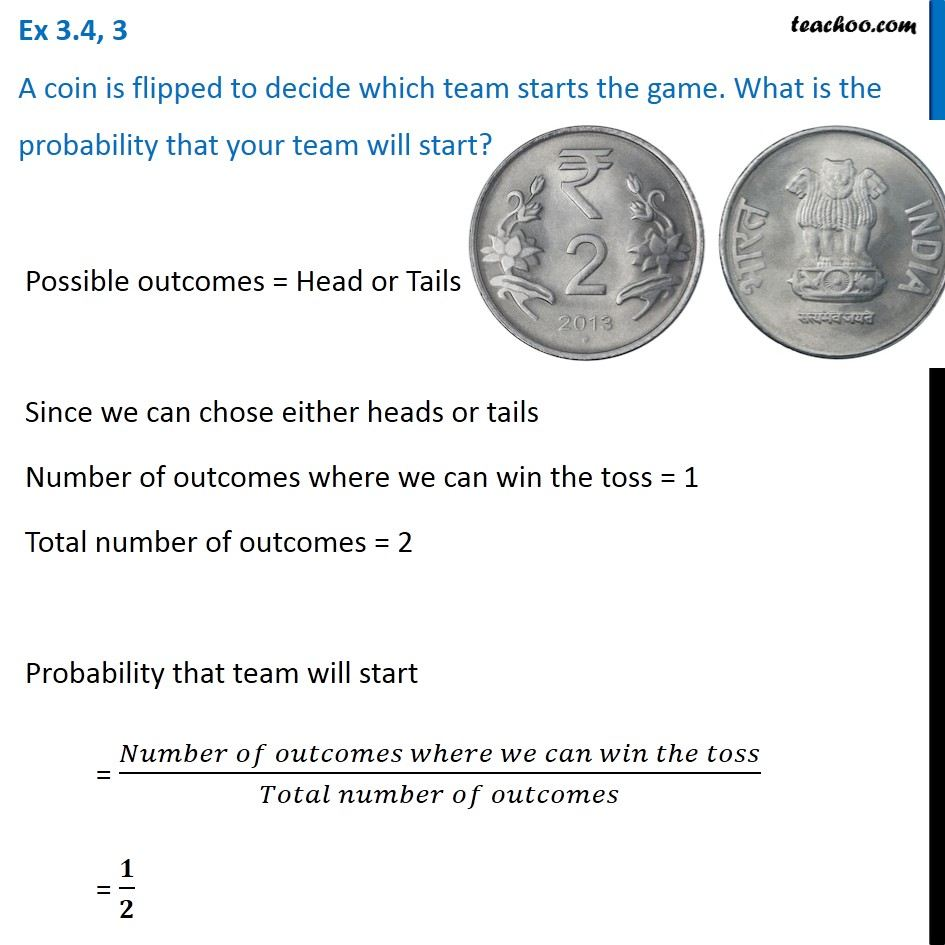1. Chapter 3 Class 7 Data Handling
2. Concept wise
3. Double Bar Graph

Transcript

Ex 3.4, 3 A coin is flipped to decide which team starts the game. What is the probability that your team will start? Possible outcomes = Head or Tails Since we can chose either heads or tails Number of outcomes where we can win the toss = 1 Total number of outcomes = 2 Probability that team will start = (𝑁𝑢𝑚𝑏𝑒𝑟 𝑜𝑓 𝑜𝑢𝑡𝑐𝑜𝑚𝑒𝑠 𝑤ℎ𝑒𝑟𝑒 𝑤𝑒 𝑐𝑎𝑛 𝑤𝑖𝑛 𝑡ℎ𝑒 𝑡𝑜𝑠𝑠)/(𝑇𝑜𝑡𝑎𝑙 𝑛𝑢𝑚𝑏𝑒𝑟 𝑜𝑓 𝑜𝑢𝑡𝑐𝑜𝑚𝑒𝑠) = 𝟏/𝟐

Double Bar Graph